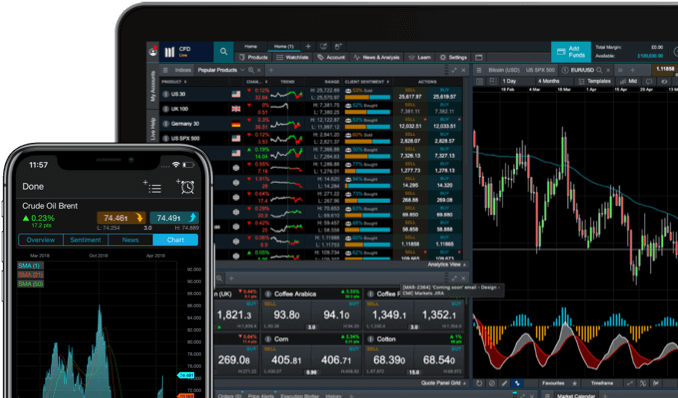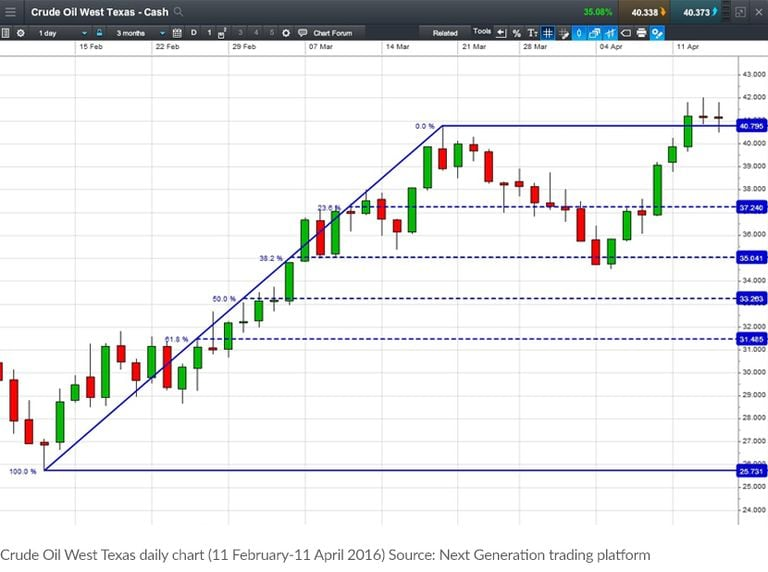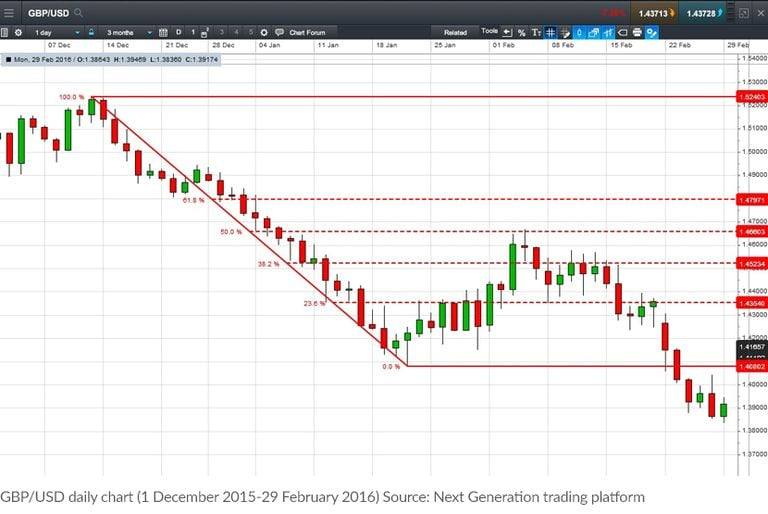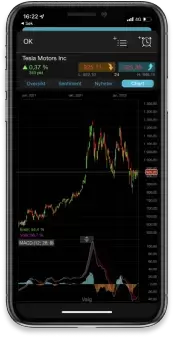I CFD sono strumenti complessi e presentano un rischio significativo di perdere denaro rapidamente a causa della leva finanziaria. Il 72% di conti di investitori al dettaglio perdono denaro a causa delle negoziazioni in CFD con questo fornitore. Valuta se comprendi il funzionamento dei CFD e se puoi permetterti di correre questo alto rischio di perdere il tuo denaro.

Fibonacci retracements are a popular form of technical analysis used by traders to predict future potential price support and resistance levels in the markets.

Scopri la nostra piattaforma di trading

Spread competitivi, nessuna commissione nascosta e 12.000 + prodottiIf used correctly, Fibonacci trends can help you identify upcoming support and resistance levels based on past price action.

Leonardo Fibonacci was a mathematician born in 1170 AD. From his work we get the Fibonacci sequence of numbers, and also the golden ratio. The Fibonacci sequence is a series of numbers where the next number is simply the sum of the two preceding numbers. So for example it would run 1, 1, 2, 3, 5, 8, 13, 21, 34, and so on.

It’s based on the rate of reproduction of two theoretical rabbits and the subsequent population growth if the following generations continued to reproduce. At first glance it may seem somewhat confusing to find there’s a connection between a 12th century mathematician, the rate at which rabbits reproduce and predicting the future direction of the financial markets using technical analysis. So why is this series of numbers so important for traders?

## Fibonacci's golden ratio

The focus tends to be on the ratio between the numbers in the sequence. It’s this that is considered to be the most important part of Fibonacci's work. Any number in the series divided by the previous number gives us 1.618 as we get further down the series. This is known as the 'golden ratio'. For Fibonacci followers there are plenty of examples in nature adhering to this ratio (or the inverse of the number, 0.618). It seems to have played an enormous part in the building blocks of everything around us.​

For example, if you divide the number of female bees by male bees in a hive you will get 1.618 as the answer. For sunflowers, each new seed is 0.618 of a turn from the last one. Fibonacci also applies to humans as well. There are lots of instances of this golden ratio working on our bodies: just one example is the ratio of the length of your forearm to your hand: 1.618.

### Fibonacci retracement levels

The argument of Fibonacci followers is: if so much of nature and the world is made up of these Fibonacci ratios, surely the same would apply to the markets too? One way in which analysts use this approach is through Fibonacci retracements. Let's say for example that a market has risen and, like all markets, it doesn't move in a straight line and starts to fall back. Traders will look at Fibonacci ratios to try and figure out where the fall may stop and the market will resume its previous rise.

### What is the Fibonacci sequence?

The golden ratio of 1.618 – that magic number – gets translated into three percentages: 23.6%, 38.2% and 61.8%. These are the three most popular percentages, although some traders will also look at the 50% and 76.4% levels. 50% is not a Fibonacci number, but has proved to be a generally popular number when correcting a primary or secondary price move. For now we will focus on the 50% and the two more popular Fibonacci percentages of 38.2% and 61.8%.

These are then applied to the chart to try and figure out potential hidden levels of support or resistance in the market. When the market drops back to 38.2% of its previous rise (the first major Fibonacci retracement), traders will check to see if any buyers come in. If this 38.2% level gets broken, then the expectation is for the 50% retracement to be the next target. If the market slides through that 50% retracement level, then traders will look to see if the market finally stops its decline when it has retraced 61.8% of the prior move. For most Fibonacci followers, if it breaks through that 61.8% level it means the market is going back to where it started.

We can create Fibonacci retracements by taking a peak and trough (or two extreme points) on a chart and dividing the vertical distance by the above key Fibonacci ratios. Once these levels are identified, horizontal lines can be drawn and then used to identify possible support and resistance levels.

Trading di CFD con un broker pluripremiato*

Prova il trading di CFD gratuitamente
e senza rischi con il nostro conto demo.

## An example of the Fibonacci pattern

This example shows the rise in the price of Crude Oil West Texas (also called WTI Crude Oil). The market then stalls, making it possible for traders to apply some Fibonacci retracements to that rally, to see where support comes in. As can be seen, the price does slide back but although briefly probing through, the 38.2% retracement in the \$35 area does end up providing some support. The market rebounds and moves out to fresh highs for the recovery.Fibonacci doesn't just apply to rising markets. If a market has fallen then Fibonacci fans will apply the retracements to the bounce back up. Let’s take an example of a market that has dropped 100 points. If it rallies 38.2% then those looking at Fibonacci retracements will expect the rally to run out of steam. If that level is broken then the 50% level is where traders would look for the market to turn back down. And finally if that one gets broken then a 61.8% retracement of the down move is the next target, with a break here suggesting the market will go all the way back to where it started the fall.

The next chart shows the drop in GBP/USD. The currency pair fell from the 1.5200 area to 1.4100, before stabilising. As the market stabilised, Fibonacci retracements could be applied to this fall. It can be seen that when the market had bounced back by 50%, the recovery ran out of steam and the slide resumed. This is an example of a Fibonacci retracement helping us to figure out when to sell short in a downtrend.Not everyone is a fan of the Fibonacci approach to market analysis. Some just see the levels as a self-fulfilling prophecy as so many people are watching them, and not having any particular 'magical' properties. But even for the sceptic, it can give an extra level of insight to potential market turning points that may not be clear at first glance.

CMC Markets è un fornitore di servizi di sola esecuzione. Queste informazioni (che contengano o meno opinioni) hanno solo scopo informativo e non tengono conto delle circostanze o degli obiettivi personali dell'utente. Nessuna delle informazioni contenute in questo documento è (o dovrebbe essere considerata) una consulenza finanziaria, di investimento o di altro tipo su cui si può fare affidamento. Nulla nei documenti costituisce una raccomandazione da parte di CMC Markets o dell'autore secondo cui un particolare investimento, titolo, transazione o strategia d'investimento sia adatto a una particolare persona. I materiali non sono stati preparati in conformità con i requisiti di legge volti a promuovere l'indipendenza della ricerca di mercato degli investimenti. Sebbene non sia espressamente vietato a noi trattare prima di fornire questo materiale, non cerchiamo di trarne profitto prima della sua diffusione.

Fai trading di CFD con CMC Markets in 3 passi

1

Invia richiesta

Completa il nostro semplice modulo di candidatura conto.

2

Deposita

Finanzia il tuo conto tramite bonifico bancario, carta di credito o PayPal.

3